Author: Oscar Cronquist Article last updated on February 07, 2022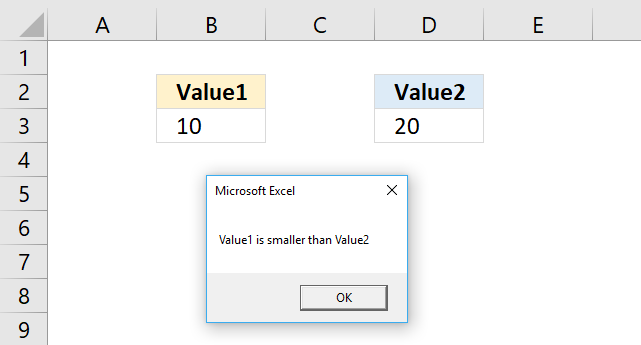This article demonstrates how to use the If ... Then statement in Visual Basic for Applications (VBA). You can combine the If ... Then statement with Else and ElseIf to make it more versatile and create more advanced conditions.

## 1. How to use the If ... then statementThe picture above demonstrates an If ... Then statement using a condition, you can use logical operators like the:

• < less than sign
• > greater than sign
• = equal sign
• <> not equal signs meaning a smaller than and a larger than sign combined.

This particular example checks if the value in B3 is smaller than the value in cell D3. If true the If statement runs the remaining code after the Then statement, in this case, it shows a message box with text Value1 is smaller than Value2. See the image above.

#### VBA code

```'Name macro
Sub Macro1()

'If ... Then statement
If Range("B3") < Range("D3") Then MsgBox "Value1 is smaller than Value2"

'Stop macro
End Sub
```

Where to put the code?

How to run a macro?

Back top

### 1.1 If ... Then condition: larger than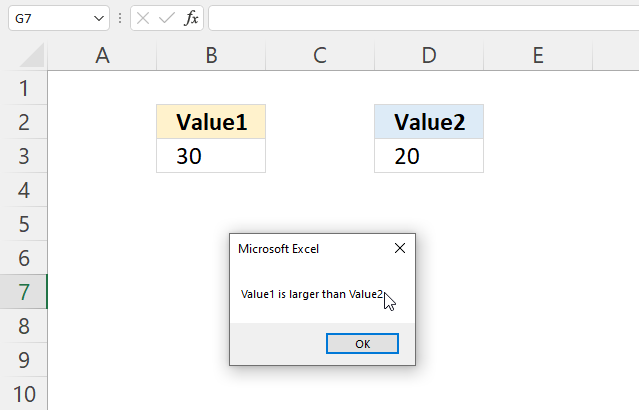#### VBA code

```'Name macro
Sub Macro1()

'If ... Then statement
If Range("B3") > Range("D3") Then MsgBox "Value1 is larger than Value2"

'Stop macro
End Sub
```

Where to put the code?

How to run a macro?

Back top

### 1.2 If ... Then condition: equal to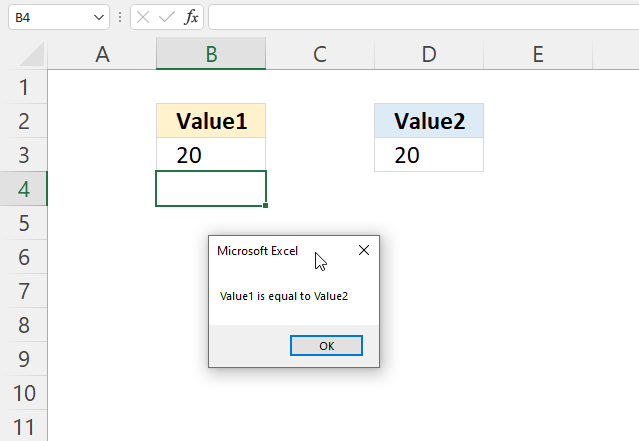#### VBA code

```'Name macro
Sub Macro1()

'If ... Then statement
If Range("B3") = Range("D3") Then MsgBox "Value1 is equal to Value2"

'Stop macro
End Sub
```

Where to put the code?

How to run a macro?

Back top

### 1.3 If ... Then condition: not equal to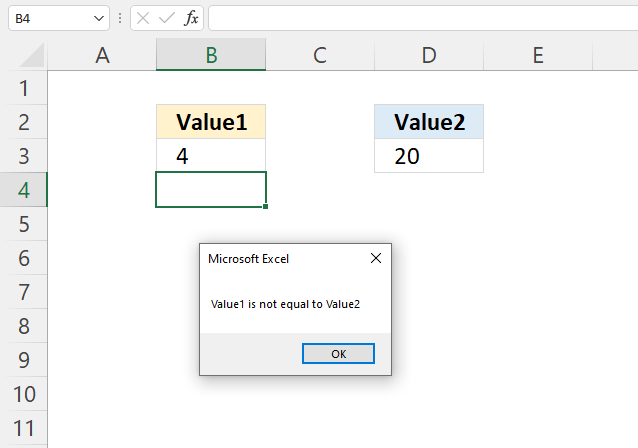#### VBA code

```'Name macro
Sub Macro1()

'If ... Then statement
If Range("B3") <> Range("D3") Then MsgBox "Value1 is not equal to Value2"

'Stop macro
End Sub
```

Where to put the code?

How to run a macro?

## 2. How to use the If ... Then ... End If statement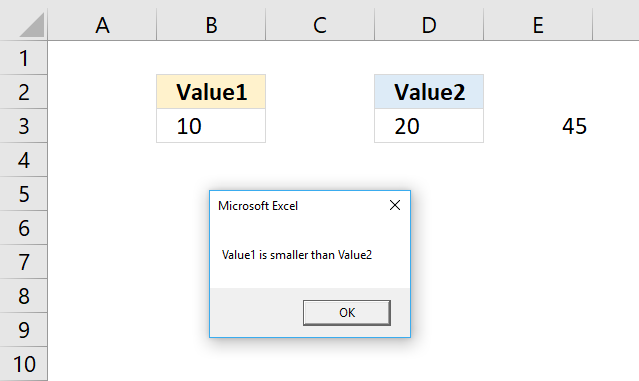The If ... Then ... End If statement allows you to run multiple lines of code, the End if statement tells the subroutine when the lines have been run and the If ... Then ... End if statement is completed.

### 2.1 VBA code

```'Name macro
Sub Macro2()

'If ... Then ... Endif statement
If Range("B3") < Range("D3") Then

'Save number 45 to cell E3 in current worksheet
Range("E3") = 45

'Show message box
MsgBox "Value1 is smaller than Value2"

End if

'Stop macro
End Sub
```

The subroutine above saves the number 45 to cell E3 if the value in cell B3 is smaller than the value in D3.

The msgbox function then displays a dialog box containing the message Value1 is smaller than Value2.

Where to put the code?

How to run a macro?

## 3. How to use the If ... Then ... Else ... End if statement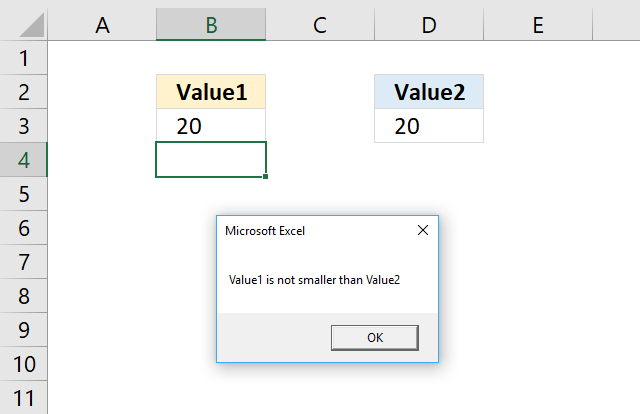The ELSE statement allows you to run code if the logical expression is not met.

### 3.1 VBA code

```'Name macro
Sub Macro3()

'If ... Then ... Else ... Endif statement
If Range("B3") < Range("D3") Then

'Display message box
MsgBox "Value1 is smaller than Value2"
Else

'Display message box
MsgBox "Value1 is not smaller than Value2"
End If

'Stop macro
End Sub
```

Where to put the code?

How to run a macro?

## 4. How to use the If ... Then ... Elseif ... Else ... Endif statement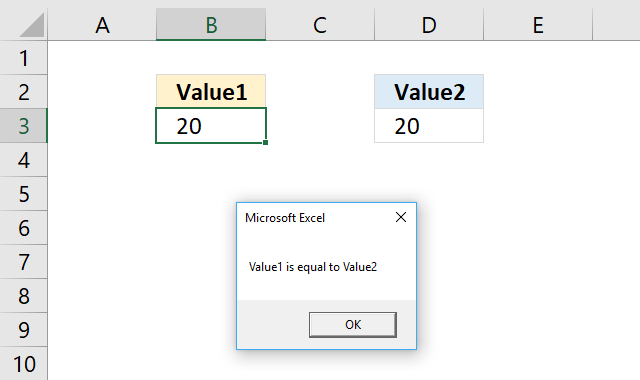The ELSEIF statement lets you create another logical expression, you may have multiple ELSEIFs in the statement.

### 4.1 VBA code

```'Name macro
Sub Macro4()
'If ... Then ... ElseIf ... Else ... Endif statement
If Range("B3") < Range("D3") Then

'Display message box
MsgBox "Value1 is smaller than Value2"
ElseIf Range("B3") = Range("D3") Then

'Display message box
MsgBox "Value1 is equal to Value2"
Else

'Display message box
MsgBox "Value1 is larger than Value2"
End If

'Stop macro
End Sub
```

Where to put the code?

How to run a macro?

## 5. Where to put the VBA code?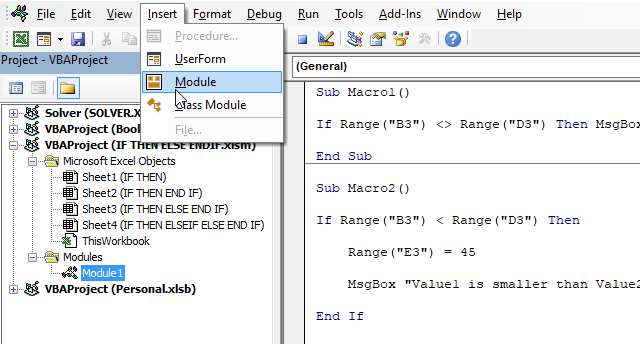1. Copy the VBA macro code.
2. Press Alt and F11 to open the Visual Basic Editor (VBE).
3. Press with left mouse button on "Insert" on the top menu.
4. Press with left mouse button on "Module", see the image above.
5. A new module is inserted, see the Project Explorer above.
6. Paste the VBA macro code to the code module.

## 6. How to run a VBA macro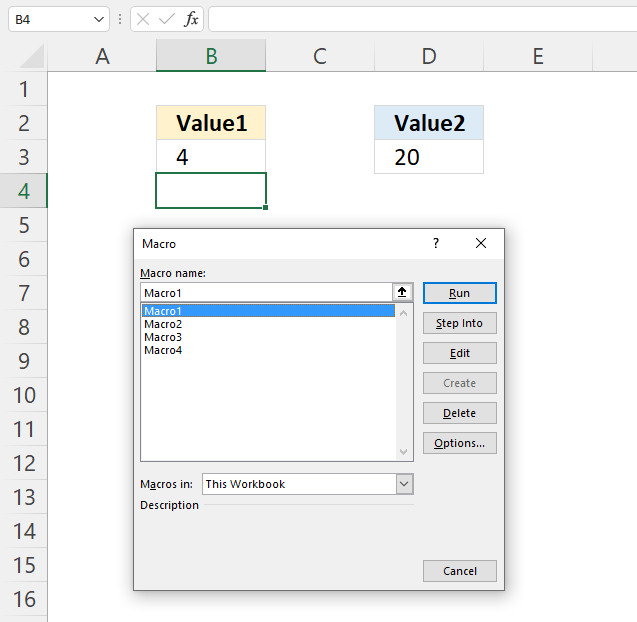1. Press Alt and F8, a dialog box appears.
2. Select the macro you want to run.
3. Press with left mouse button on "Run" button.

## Get Excel *.xlsm macro-enabled file

IF THEN ELSE ENDIF.xlsm